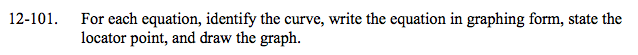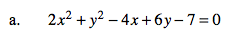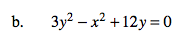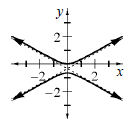### Home > A2C > Chapter 12 > Lesson 12.2.1 > Problem12-101

12-101.Complete the squares.

2(x2 − 2x + 1) − 2 + (y2 + 6y + 9) − 9 − 7 = 0Complete the squares. Notice that the x2 term is subtracted.